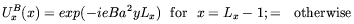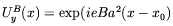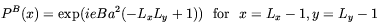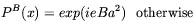5.1.8. Proton neutron mass difference by a lattice calculation

One can take another approach, in principle exact, to calculate the mass difference using lattice gauge theory. We introduce the magnetic field in the lattice by multiplying a link Uµ(x) with a phase UµB(x). Fixing the field,for convenience, in the direction z we then set:(5.35)(5.36)

where x0 is an offset for the magnetic field.

Consequently the plaquette in the x-y plane is(5.37)(5.38)

The magnetic field is homogeneous only if B is is quantized as a2 eB = 2n(LxLy)-1 This is a troublesome condition since the field is very large for reasonable lattice size. In the simulations we ignored this condition inducing some inhomogeneity. The results are very preliminary: at this stage it is difficult to find these effects with present lattice technology, but in principle it is possible. For details of the calculations see . This method is still not efficient given the present state of the art in simulations.